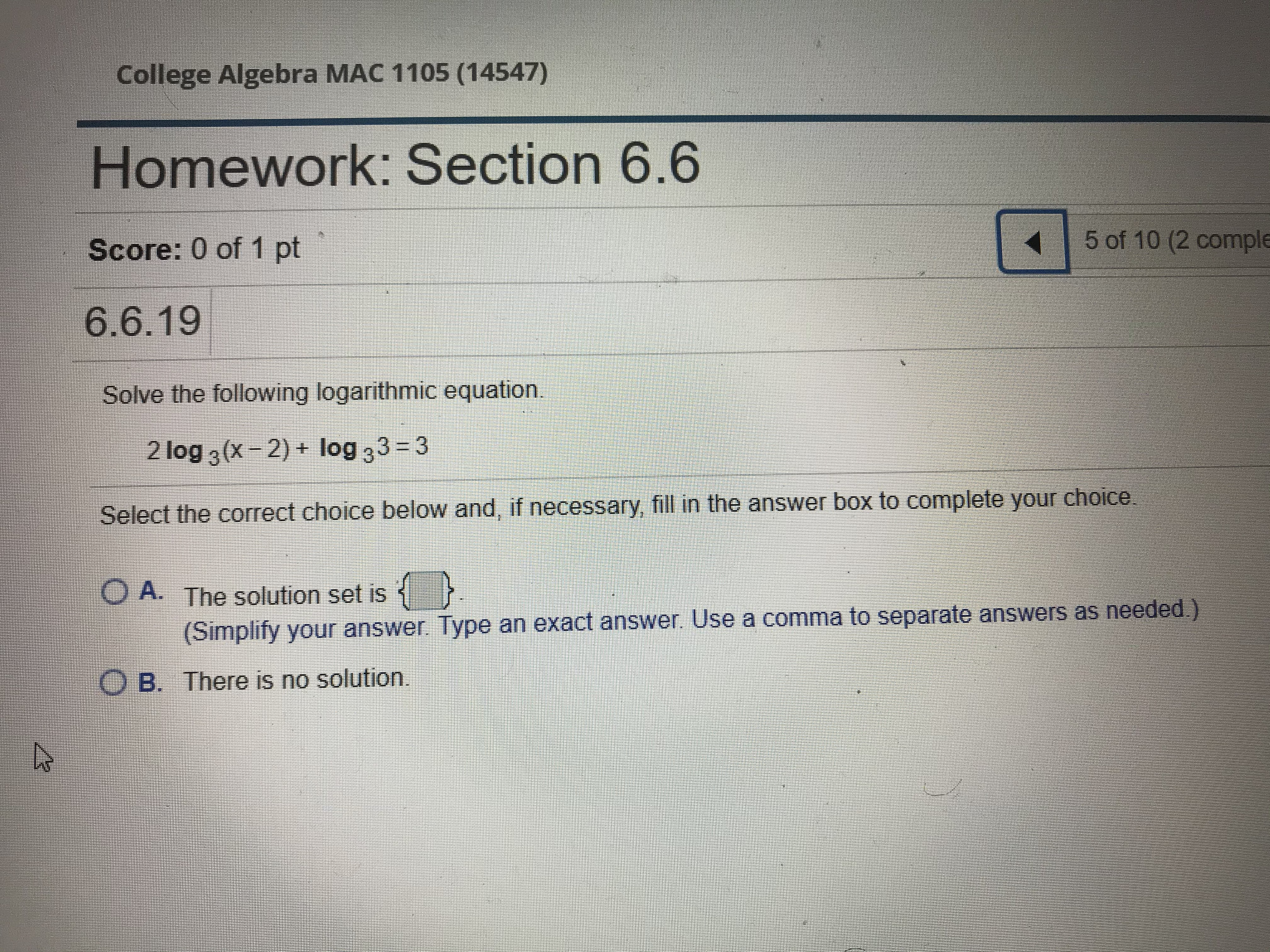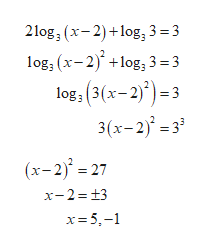# College Algebra MAC 1105 (14547)Homework: Section 6.65 of 10 (2 compleScore: 0 of 1 pt6.6.19Solve the following logarithmic equation.2 log 3 (x-2)+ log 33 3Select the correct choice below and, if necessary, fill in the answer box to complete your choiceO A. The solution set is(Simplify your answer. Type an exact answer. Use a comma to separate answers as needed.)O B. There is no solution.

Question
24 views
Solve the following logarithmic equation.

2 log Subscript 3 Baseline left parenthesis x minus 2 right parenthesis plus log Subscript 3 Baseline 3 equals 32log3(x−2)+log33=3
Select the correct choice below​ and, if​ necessary, fill in the answer box to complete your choice.help_outlineImage TranscriptioncloseCollege Algebra MAC 1105 (14547) Homework: Section 6.6 5 of 10 (2 comple Score: 0 of 1 pt 6.6.19 Solve the following logarithmic equation. 2 log 3 (x-2)+ log 33 3 Select the correct choice below and, if necessary, fill in the answer box to complete your choice O A. The solution set is (Simplify your answer. Type an exact answer. Use a comma to separate answers as needed.) O B. There is no solution. fullscreen
check_circle

Take the terms outside the logarithm into the argument and t...help_outlineImage Transcriptionclose2log3 (x-2)log3 3 =3 log, (x-2)+log, 3= 3 log, (3(x-2)3 3(x-2j' =3 (x-2)27 x-2 13 x- 5- fullscreen

### Want to see the full answer?

See Solution

#### Want to see this answer and more?

Solutions are written by subject experts who are available 24/7. Questions are typically answered within 1 hour.*

See Solution
*Response times may vary by subject and question.
Tagged in
MathAlgebra

### Equations and In-equations